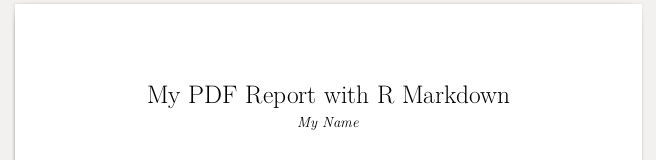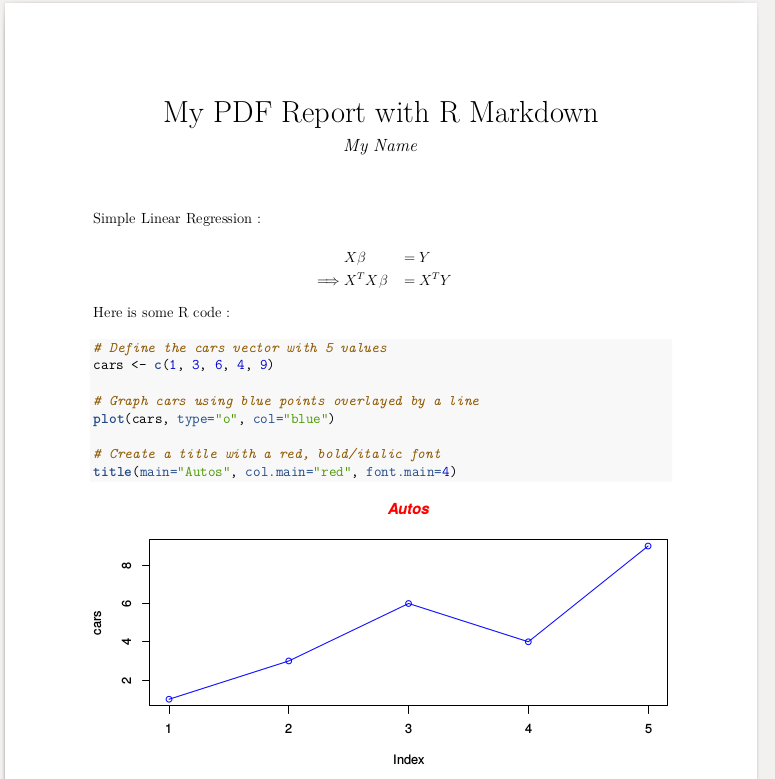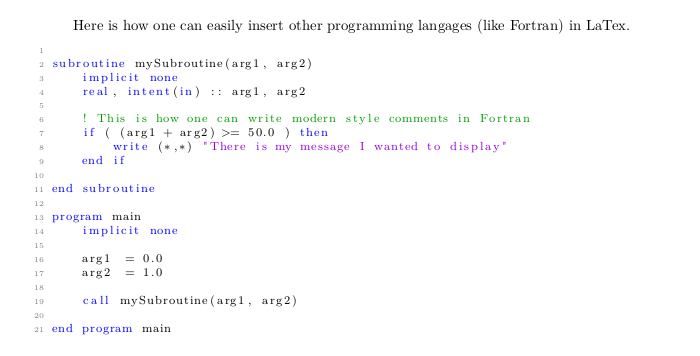# October 2017

## Introduction

Today, I will be talking about how to generate a nice pdf report with text, code, plots, and formulas using R markdown.

For those of you who are in a hurry, you will find the entire code at the end of this post. Simply skip to Step 2 to learn how to convert it into pdf.

Once again, I will explain how to do this in command line. Because why would anyone need graphical interfaces when they have Vim ?

## Step 1 : Create a basic .Rmd file

Save the following lines in a file named, say, “my_report.Rmd” :

---
title: "My PDF Report with R Markdown"
author:
- First Name, Last Name
output: pdf_document
fontsize: 12pt
---


## Step 2 : Convert .Rmd -> PDF

Command line in the same directory

>> Rscript -e “rmarkdown::render(‘./my_report.Rmd’)”

You sould find a file named my_report.pdf in the same directory.

The file should look like this :## Step 3 : Add some text, and a formula

Simple Linear Regression :

\begin{aligned} &X \beta &&= Y \\ \implies &X^{T} X \beta &&= X^{T} Y \\ \end{aligned}


Step 2 to update the PDF

Formulas are written using LaTex formatting.

## Step 4 : Add some R code, and a plot

Here is some R code :

{r}
# Define the cars vector with 5 values
cars <- c(1, 3, 6, 4, 9)

# Graph cars using blue points overlayed by a line
plot(cars, type="o", col="blue")

# Create a title with a red, bold/italic font
title(main="Autos", col.main="red", font.main=4)



Step 2 to update the PDF

More “generic” plot ideas here.

## Step 5 : Change the plot size

In the above code, change

{r}

for

{r, fig.width=8, fig.height=4}


Step 2 to update the PDF

## Step 5 : Summary

Here is the entire sample code and the resulting PDF you can expect to have.

---
title: "My PDF Report with R Markdown"
author:
- My Name
output: pdf_document
fontsize: 12pt
---

Simple Linear Regression :

\begin{aligned} &X \beta &&= Y \\ \implies &X^{T} X \beta &&= X^{T} Y \\ \end{aligned}

Here is some R code :

{r, fig.width=8, fig.height=4}
# Define the cars vector with 5 values
cars <- c(1, 3, 6, 4, 9)

# Graph cars using blue points overlayed by a line
plot(cars, type="o", col="blue")

# Create a title with a red, bold/italic font
title(main="Autos", col.main="red", font.main=4)## LaTeX PDF’s with Fortran Source Code Highlighting

Oh My God she is back with her FORTRAN thing again …

Why does she keep showing us how to do this and that in FORTRAN …

Why can’t she do R and Python just like everybody else …

Don’t get me wrong I do code in R and Python most of the time. It’s just I figured out there must be at least tens of people on this planet who will wonder someday, just like I did last month : “How do I generate a nice LaTeX PDF report with FORTRAN code in it ?”.

Well, I suppose you run Fortran on Linux.

### Step 1: Write the .tex file

\documentclass{article}

\usepackage[T1]{fontenc}
\usepackage{xcolor}
\usepackage{lmodern}
\usepackage{listings}
\usepackage[margin=1.0in]{geometry}

\definecolor{mygreen}{rgb}{0,0.6,0}
\definecolor{mygray}{rgb}{0.5,0.5,0.5}
\definecolor{mymauve}{rgb}{0.58,0,0.82}

\lstset{
basicstyle=\footnotesize,        % the size of the fonts that are used for the code
breakatwhitespace=false,         % sets if automatic breaks should only happen at whitespace
breaklines=false,                 % sets automatic line breaking
captionpos=b,                    % sets the caption-position to bottom
extendedchars=true,              % lets you use non-ASCII characters; for 8-bits encodings only, does not work with UTF-8
keepspaces=true,                 % keeps spaces in text, useful for keeping indentation of code (possibly needs columns=flexible)
keywordstyle=\color{blue},       % keyword style
language=Fortran,                 % the language of the code
numbers=left,                    % where to put the line-numbers; possible values are (none, left, right)
numbersep=5pt,                   % how far the line-numbers are from the code
numberstyle=\tiny\color{mygray}, % the style that is used for the line-numbers
rulecolor=\color{black},         % if not set, the frame-color may be changed on line-breaks within not-black text (e.g. comments (green here))
showspaces=false,                % show spaces everywhere adding particular underscores; it overrides 'showstringspaces'
showstringspaces=false,          % underline spaces within strings only
showtabs=false,                  % show tabs within strings adding particular underscores
stepnumber=1,                    % the step between two line-numbers. If it's 1, each line will be numbered
stringstyle=\color{mymauve},     % string literal style
tabsize=4,                       % sets default tabsize to 2 spaces
title=\lstname                   % show the filename of files
}

\begin{document}

Here is how one can easily insert other programming langages (like Fortran) in LaTex.

\begin{lstlisting}

subroutine mySubroutine()

write (*,*) "Hello"
end subroutine

program main
implicit none

call mySubroutine()

end program main

\end{lstlisting}

\end{document}

The rules inside \lstset {} will configure your syntax highlighting. You may change them according to your own taste.

I also mentioned that language=Fortran, but you may also write language=Fortran if you like it more old school.

Then save your file as, say, “example.tex”.

### Step 2: Install the necessary packages

>>> sudo apt-get install texlive-full



### Step 3 :  Generate the pdf

>>> pdflatex ./example.tex

`

### Step 4 :  Open the pdf